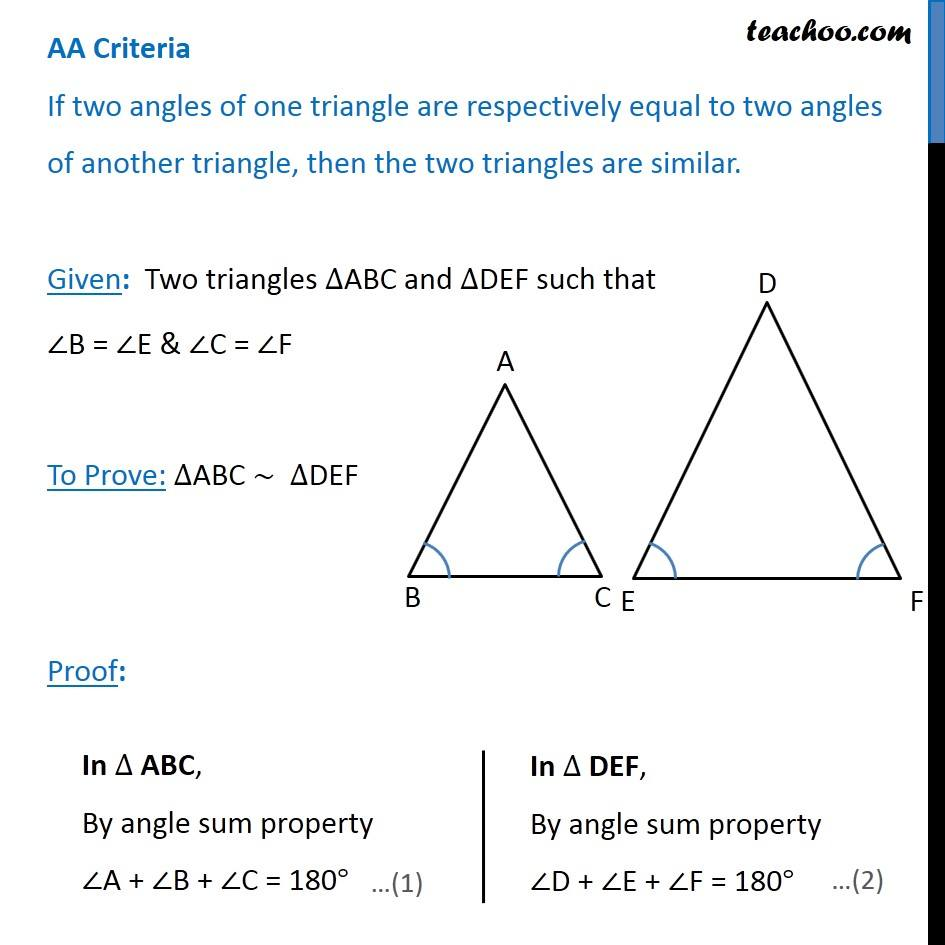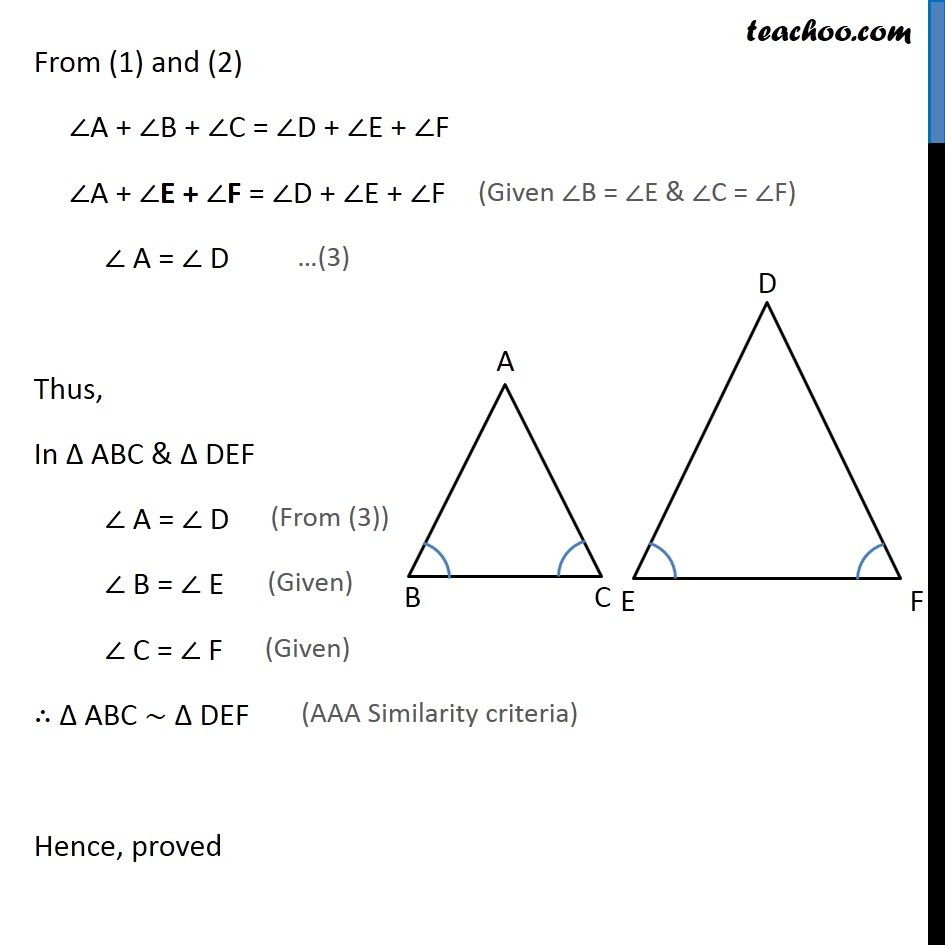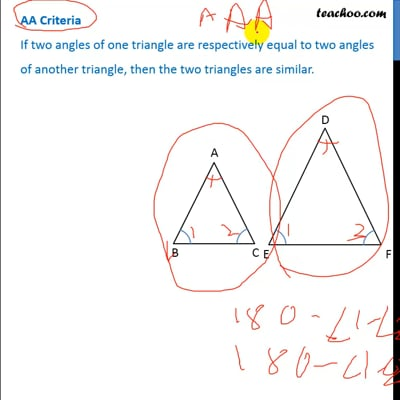Theorems

Chapter 6 Class 10 Triangles
Serial order wiseThis video is only available for Teachoo black users

Learn in your speed, with individual attention - Teachoo Maths 1-on-1 Class

### Transcript

AA Criteria If two angles of one triangle are respectively equal to two angles of another triangle, then the two triangles are similar.Given: Two triangles ∆ABC and ∆DEF such that ∠B = ∠E & ∠C = ∠F To Prove: ∆ABC ~ ∆DEF Proof: In ∆ ABC, By angle sum property ∠A + ∠B + ∠C = 180° In ∆ DEF, By angle sum property ∠D + ∠E + ∠F = 180° In ∆ DEF, By angle sum property ∠D + ∠E + ∠F = 180° From (1) and (2) ∠A + ∠B + ∠C = ∠D + ∠E + ∠F ∠A + ∠E + ∠F = ∠D + ∠E + ∠F ∠ A = ∠ D Thus, In Δ ABC & Δ DEF ∠ A = ∠ D ∠ B = ∠ E ∠ C = ∠ F ∴ Δ ABC ~ Δ DEF Hence, proved